Ozma of Oz

Plaintext for L. Frank Baum's "Ozma of Oz"

Examples

Basic Examples

Retrieve the resource:

 In:=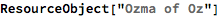Out=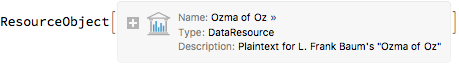Retrieve the default content:

 In:=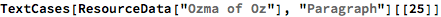Out=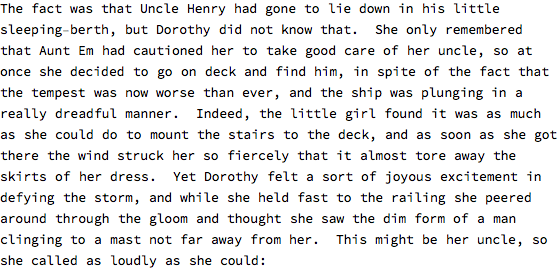Visualization

Create a word cloud:

 In:=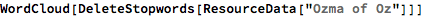Out=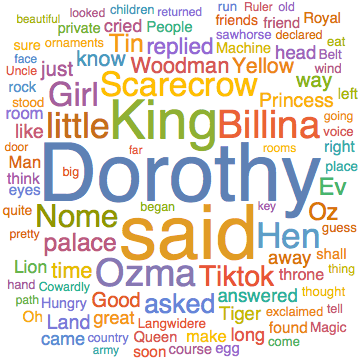Wolfram Research, "Ozma of Oz" from the Wolfram Data Repository (2016) https://doi.org/10.24097/wolfram.12872.data